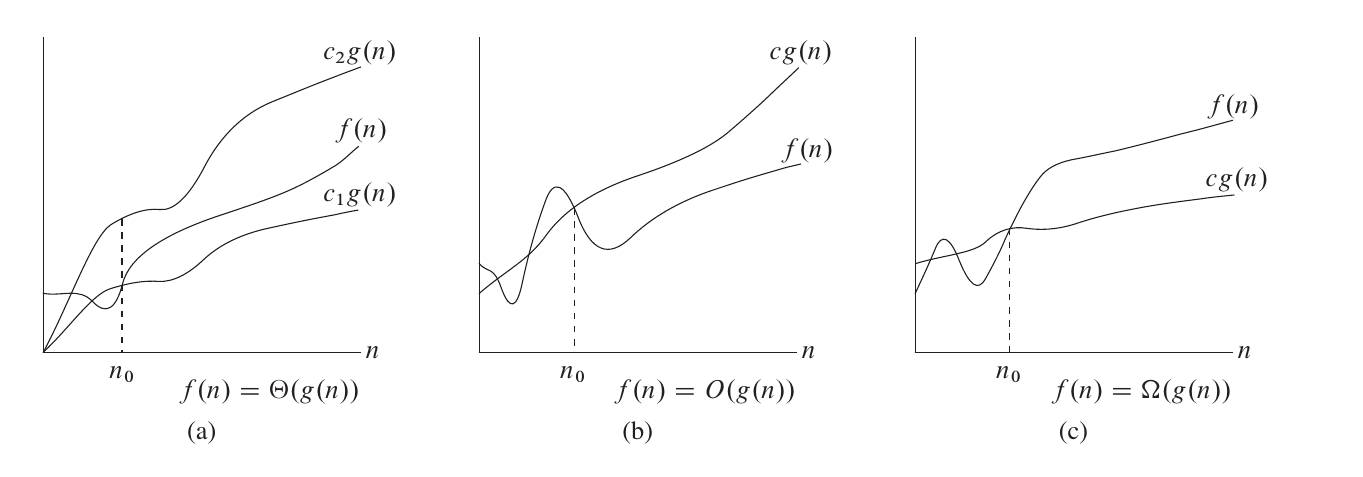### 第一章 引言

• 连通性问题：
• 两个操作：查找（find）、并集（union）
• 快速-查找（quick-find）：N 个对象，M 次合并：MN
• 快速-合并（quick-union）：N 个对象，M 对：MN/2
• 加权快速-合并（weighted quick-union）：遍历 2lgN 个指针：线性
• 带等分路径压缩的加权快速-合并：保证线性
• 在线算法（online）：能处理的数据没有限制

### 第二章 算法分析的原理• 算法分析的种类：
• 最坏情况（Worst Case）：任意输入规模的最大运行时间（Usually）
• 在任何输入下运行时间的一个上界
• 平均情况（Average Case）：任意输入规模的期待运行时间（Sometimes）
• 最佳情况（Best Case）：通常最佳情况不会出现（Bogus）
• 基本思路：
• 忽略掉那些依赖于机器的常量
• 关注运行时间的增长趋势
• 渐近记号：
• 尽管技术上 theta 符号较为准确，但通常仍然使用大 O 符号表示
• 大 O 符号：只有渐近上界
• 表示最坏运行情况的上界
• T(n) = O(n^3) 等同于 T(n) ∈ O(n^3)：T(n) 的渐近增长不快于 n^3
• theta（西塔）符号：渐近地给出了一个函数的上界和下界
• T(n) = Θ(n^3) 等同于 T(n) ∈ Θ(n^3)：T(n) 的渐近增长与 n^3 一样快
• omega（欧米伽）符号：只有渐近下界
• 采用记号 lgN = log_2N：改变一个对数的底只是把对数的值改变了一个常数倍，所以当不在意这些常数因子时可使用这个简便的记法
• 常见复杂度：
• 常量：O(1)
• 对数：O(lgN)
• 线性：O(N)
• 线性对数：O(NlgN)
• 平方：O(N^2)
• 立方：O(N^3)
• 指数：O(2^N) O(k^N)
• 阶乘：O(N!)：旅行商问题
• floor(x)：向下取整 x。设 x = 4.5，floor(x) = 4
• ceil(x)：向上取整 x。设 x = 4.5，ceil(x) = 5
• 调和级数：H_N = 1 + 1/2 + 1/3 + … + 1/N